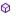# Android Question"true","false",True, False How do they work?

#### jtare

##### Active Member
Longtime User
I noticed that for some reason a boolean stored in a map that was read from a file no longer is a boolean but a string maybe?

I ran some tests where mymap.get("a") is False and mymap.get("b") is False and got this:
B4X:
``````    Dim mymap As map = File.ReadMap(File.DirInternal,"mymap.txt")
Log(Not(mymap.Get("a") Or mymap.Get("b"))) 'True
Log("false"=False) 'True
Log("false"=True) 'False
Log("true"=True) 'True
Log(mymap.Get("a") = False And mymap.Get("b") = False) 'False
Log(mymap.Get("a") = False) 'False
Log(mymap.Get("b") = False) 'False
Log(mymap.Get("a") = "false" And mymap.Get("b") = "false") 'True
Log(mymap.Get("a") = "false") 'True
Log(mymap.Get("b") = "false") 'True``````

Both "a" and "b" value are set with a boolean variable, not string. Like this:
B4X:
``mymap.put("a",False)``
I used to store the map in the keyvaluestore database and worked as expected. This weird behavior started when I moved to save it and read it from a file.

If the mymap is no longer holding a boolean but a string, shouldn't
B4X:
``log(mymap.Get("a") = False ) 'False``
and
B4X:
``log("false"=False) 'True``
Be the same? Both True? But instead I get False in the first one and True in the second one.

#### DonManfred

##### Expert
Longtime UserFile. WriteMap (Dir As String, FileName As String, Map As Map)

Creates a new file and writes the given map. Each key value pair is written as a single line.
All values are converted to strings. <--- !!!

You should use B4ASerialisator or Randomaccessfile WriteB4XObject/ReadB4XObject

•José J. Aguilar and jtare

#### jtare

##### Active Member
Longtime User
You should use B4ASerialisator or Randomaccessfile WriteB4XObject/ReadB4XObject
Thanks, I'll give it a try.

But still shoudn't
B4X:
``log(mymap.Get("a") = False ) 'False``
and
B4X:
``log("false"=False) 'True``
Be the same?

#### DonManfred

##### Expert
Longtime User
Cast the string to a Boolean and compare the two booleans.

•jtare

#### Computersmith64

##### Well-Known Member
Longtime User
I use ReadMap & WriteMap a lot in my apps to save settings, so for Booleans I just use this utility function:

B4X:
``````Private Sub CBool(sVal As String) As Boolean
If sVal = "true" Then Return True Else Return False
End Sub``````

- Colin.

•mangojack and José J. Aguilar

#### jtare

##### Active Member
Longtime User
I use ReadMap & WriteMap a lot in my apps to save settings, so for Booleans I just use this utility function:

B4X:
``````Private Sub CBool(sVal As String) As Boolean
If sVal = "true" Then Return True Else Return False
End Sub``````

- Colin.
Thanks for the suggestion. I think I will go with this solution for now.

#### RB Smissaert

##### Well-Known Member
Longtime User
I use ReadMap & WriteMap a lot in my apps to save settings, so for Booleans I just use this utility function:

B4X:
``````Private Sub CBool(sVal As String) As Boolean
If sVal = "true" Then Return True Else Return False
End Sub``````

- Colin.

Not sure it is any better, but I would do:

B4X:
``Return sVal = "true"``

or maybe better

B4X:
``Return sVal.ToLowerCase = "true"``

RBS

•jtare

#### klaus

##### Expert
Longtime User
B4X:
``````Private Mapa, Mapb As Boolean
Mapa = MyMap.Get("a")
Mapb = MyMap.Get("b")``````
This works.
The problem is that MyMap.Get("a") is a String.
But, if you set MyMap.Get("a") to a Boolean variable, like Mapa = MyMap.Get("a"), B4A sets the value correctly.

•jtare

#### Cableguy

##### Expert
Longtime User
Why not "simplify" things and set True=1 and false = 0, the IDE would then convert the string to the correct type, would it not?

•vecino and jtare

#### jtare

##### Active Member
Longtime User
B4X:
``````Private Mapa, Mapb As Boolean
Mapa = MyMap.Get("a")
Mapb = MyMap.Get("b")``````
This works.
The problem is that MyMap.Get("a") is a String.
But, if you set MyMap.Get("a") to a Boolean variable, like Mapa = MyMap.Get("a"), B4A sets the value correctly.
Thanks.

Why not "simplify" things and set True=1 and false = 0, the IDE would then convert the string to the correct type, would it not?
Will have to check if "0"=0 is the same to mymap.get("a")=0, if not it kind of leave me in the same situation.